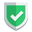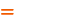我们相信：世界是美好的，你是我也是。平行空间的世界里面，不同版本的生活也在继续...

## 更新节点标签

``match(n:Person) set n:Human``

``match(n:Human) set n:人类 remove n:Human``

``match(n:Person) remove n:人类``

## 更新节点属性

``Match(n:Person{name:'sunan'}) set n.name='sunan',n.born=2000,n.live='北京'``

``Match(n:Person{name:'sunan'}) remove n.born return n``

``Match(n:Person{name:'sunan'}) set n.生活在=n.live remove n.live return n``

## 建立新的关系

``````MATCH (a),(b) WHERE a.name="sunan" AND b.title="The Matrix" CREATE (a) -[r:Watch {count: 1}]-> (b) return r;
match (n:Person{name:'sunan'})-[r]->(m:Movie{title:'The Matrix'}) return type(r);``````

## 更新或添加关系属性

``match (n:Person{name:'sunan'})-[r]->(m:Movie{title:'The Matrix'}) set r.aa="aaa" return r,type(r)``

## 删除关系属性

``````match (n:Person{name:'sunan'})-[r]->(m:Movie{title:'The Matrix'})
remove r.aa
return r,type(r)``````

## 更新关系类型

``````MATCH (n:Person{name:'sunan'})-[r:Watch]->(m:Movie{title:'The Matrix'})
CREATE (n)-[r2:Learn]->(m)
SET r2 = r
WITH r
DELETE r``````

## 综述如果本文对您有帮助，或者节约了您的时间，欢迎打赏瓶饮料，建立下友谊关系。本博客不欢迎：各种镜像采集行为。请尊重原创文章内容，转载请保留作者链接。【福利】 腾讯云最新爆款活动！1核2G云服务器首年50元！【源码】本文代码片段及相关软件，请点此获取更多信息【绝密】秘籍文章入口，仅传授于有缘之人neo4j    cypher